Courses

# TEST: Addition (Give And Take) - 2

## 10 Questions MCQ Test Mathematics (Maths) for Class 3 | TEST: Addition (Give And Take) - 2

Description
This mock test of TEST: Addition (Give And Take) - 2 for Class 3 helps you for every Class 3 entrance exam. This contains 10 Multiple Choice Questions for Class 3 TEST: Addition (Give And Take) - 2 (mcq) to study with solutions a complete question bank. The solved questions answers in this TEST: Addition (Give And Take) - 2 quiz give you a good mix of easy questions and tough questions. Class 3 students definitely take this TEST: Addition (Give And Take) - 2 exercise for a better result in the exam. You can find other TEST: Addition (Give And Take) - 2 extra questions, long questions & short questions for Class 3 on EduRev as well by searching above.
QUESTION: 1

Solution:

20+30=15+35=50

QUESTION: 2

### Match the following: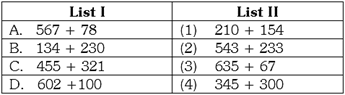Solution:

567 + 78 = 645 = 345 + 300 134 + 230 = 364 = 210 + 154 455 + 321 = 776 = 543 + 233 602 + 100= 702 = 635 + 67

QUESTION: 3

### Match the following: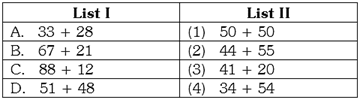Solution:

33 + 28 = 61 = 41 + 20 67 + 21 = 88 = 34 + 54 88 + 12 = 100 = 50 + 50 51 + 48 = 99 = 44 + 55

QUESTION: 4

The Venn diagram below shows the number of students who voted for their favorite sport. How many students voted in all?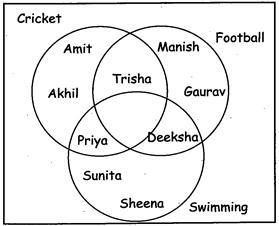Solution:

Count the names of students in the diagram to calculate the total number of students who voted.

QUESTION: 5

The diagram below shows how class III students voted for their favorite pet How many total number of students want dog or fish as their pet? Favorite Pet of Class III students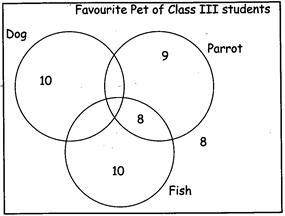Solution:

Number of students who want dog as their pet = 10 Number of students who want Fish as their pet = 10+ 8 = 18 10 + 18 = 28.

QUESTION: 6

Which numbers complete the pattern? 4 + 5= ___ 40 + 50 = ___ 400 + 500 = ____ 4000 + 5000 = ____

Solution:

4+5 = 9 40+50 = 90 400 + 500 = 900 4000 + 5000 = 9000

QUESTION: 7

Which numbers complete the pattern? ___ +6=8 ___ + 60= 80 ___ + 600= 800

Solution:

8 ? 6 = 2 80 ? 60 = 20 800 ? 600 = 200

QUESTION: 8

Look at the table below. What is rule for the table?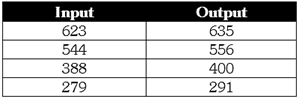Solution:

623 + 12 = 635 544 + 12 = 556 388 + 12 = 400 279 + 12 = 291

QUESTION: 9

Look at the table below. What is rule for the table?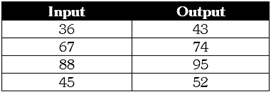Solution:

36 + 7 = 43 67 + 7 =74 88 + 7 = 95 45 + 7 = 52

QUESTION: 10

Fill in the table below. The rule is to Add 40 to each term.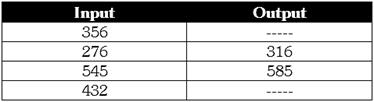Solution:

356 + 40 = 396 432 + 40 = 472